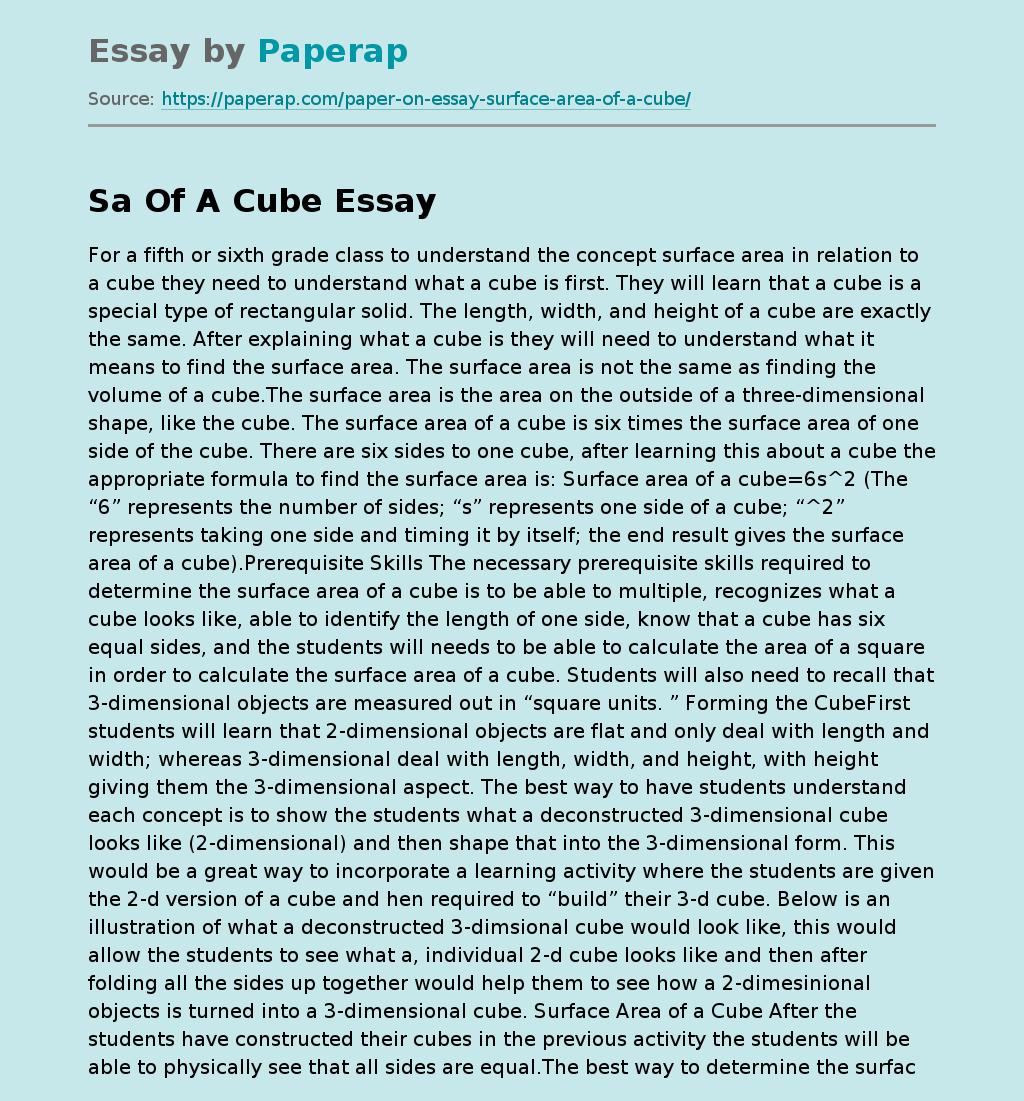# Understanding Surface Area of a Cube

For a fifth or sixth grade class to understand the concept surface area in relation to a cube they need to understand what a cube is first. They will learn that a cube is a special type of rectangular solid. The length, width, and height of a cube are exactly the same. After explaining what a cube is they will need to understand what it means to find the surface area. The surface area is not the same as finding the volume of a cube.

The surface area is the area on the outside of a three-dimensional shape, like the cube. The surface area of a cube is six times the surface area of one side of the cube. There are six sides to one cube, after learning this about a cube the appropriate formula to find the surface area is: Surface area of a cube=6s^2 (The “6” represents the number of sides; “s” represents one side of a cube; “^2” represents taking one side and timing it by itself; the end result gives the surface area of a cube).

Prerequisite Skills The necessary prerequisite skills required to determine the surface area of a cube is to be able to multiple, recognizes what a cube looks like, able to identify the length of one side, know that a cube has six equal sides, and the students will needs to be able to calculate the area of a square in order to calculate the surface area of a cube. Students will also need to recall that 3-dimensional objects are measured out in “square units.

Get quality help nowWriterBelleVerified

Proficient in: Communication4.7 (657)

“ Really polite, and a great writer! Task done as described and better, responded to all my questions promptly too! ”+84 relevant experts are online

” Forming the CubeFirst students will learn that 2-dimensional objects are flat and only deal with length and width; whereas 3-dimensional deal with length, width, and height, with height giving them the 3-dimensional aspect. The best way to have students understand each concept is to show the students what a deconstructed 3-dimensional cube looks like (2-dimensional) and then shape that into the 3-dimensional form. This would be a great way to incorporate a learning activity where the students are given the 2-d version of a cube and hen required to “build” their 3-d cube. Below is an illustration of what a deconstructed 3-dimsional cube would look like, this would allow the students to see what a, individual 2-d cube looks like and then after folding all the sides up together would help them to see how a 2-dimesinional objects is turned into a 3-dimensional cube. Surface Area of a Cube After the students have constructed their cubes in the previous activity the students will be able to physically see that all sides are equal.The best way to determine the surface area would be to ask them how much material they think it would take to cover the cube completely. After learning that the cube has six sides and all sides are equal I would tell them that one side is equal to 4 and to have them determine, based off the formula they have learned to tell me how much material, or how much surface area there is to cover. Below is what the formula would look like: ·Surface area of a cube=6s^2 ·Surface area of a cube=6(4)^2 ·Surface area of a cube=6(16) Surface area of a cube=96 square units (3-d objects are measured in square units). ·After giving them the length of one side of their cube, the students are able to calculate the above formula to determine the surface area is 96 square units. Students will require much more practice to master this skill. After completing the above I will give them a worksheet with 10 or so different size cubes with one side given and they will need to plug that into the given formula to determine the surface area.Another activity to test competency would be to have them bring in one real life object from home, or to find one around the room that represents a cube so that I may gauge their understanding in what a cube is and have them measure one side and compute the overall surface area. This could be used in addition to the end of the week test. References Long, Lynette. (2009). Painless geometry. Hauppauge, New York: Barron’s Educational Series, Inc. Lorandini, Caryl. (2012). E-Z pre-algebra. Hauppauge, New York: Barron’s Educational Series, Inc.